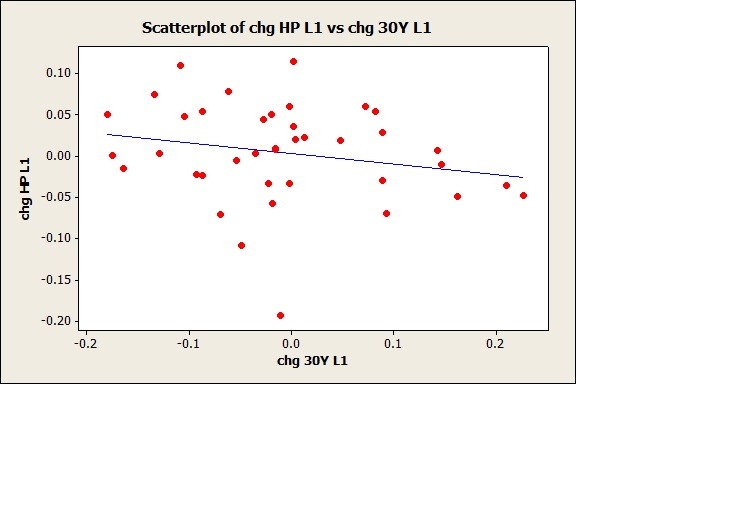### Sep

#### 17

September 17, 2012 |

Monthly 30Y fixed mortgage rates are published by Freddie Mac, 1971-present: http://www.freddiemac.com/pmms/pmms30.htm

This data was used to calculate mean 30Y mortgage rates by year, 1971-2011. Prof Shiller's quarterly real US house price data was also used to calculate mean yearly real house price, 1971-2011.

These data were then analyzed with regression; first regressing this year's change in real house price vs this year's change in 30Y mortgage rate:

The regression equation is
chg HP = 0.00393 + 0.0108 chg 30Y

Predictor      Coef   SE Coef       T      P
Constant    0.00393  0.009442  0.42  0.679
chg 30Y     0.01080   0.09513  0.11  0.910

S = 0.0595077   R-Sq = 0.0%   R-Sq(adj) = 0.0%

There was no correlation between this year's change in real house price vs this year's change in 30Y mortgage rate.

Is there a correlation with a lagged regression? This year's change in real house price was regressed against last year's change in 30Y mortgage rate:

Regression Analysis: chg HP L1 versus chg 30Y L1

The regression equation is
chg HP L1 = 0.00281 - 0.127 chg 30Y L1

Predictor        Coef      SE Coef       T      P
Constant       0.00281   0.00945   0.30  0.768
chg 30Y L1  -0.12724   0.09436  -1.35  0.186

S = 0.0588827   R-Sq = 4.7%   R-Sq(adj) = 2.1%

Not significant, but as one might expect if last year's mortgage rates dropped, this year's house prices increased slightly (see scatter plot).

Evidently there is a weak effect of mortgage rates on real house prices

Just for fun:

The Zombies- Time of the Season [video]

The Zombies- She's Not There[video]`SELECT * FROM wp_comments WHERE comment_post_ID = '7712' AND comment_approved = '1' ORDER BY comment_date`